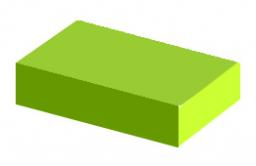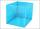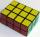# Cuboid surface

Determine surface area of cuboid if its volume is 52.8 cm cubic and length of the two edges are 2 cm and 6 cm.

S =  94.4 cm2

### Step-by-step explanation:Did you find an error or inaccuracy? Feel free to write us. Thank you!Tips to related online calculators
Tip: Our volume units converter will help you with the conversion of volume units.

## Related math problems and questions:

• Surface of cuboidFind the surface of the cuboid if its volume is 52.8 cm3 and the length of its two edges is 2 cm and 6 cm.
• Cuboid - VabFind the surface of the cuboid when its volume is 52.8 cubic centimeters, and the length of its two edges is 2 centimeters and 6 centimeters.
• Cube 5The content area of one cube wall is 32 square centimeters. Determine the length of its edges, its surface and volume.
• CuboidCuboid has a surface of 516 cm2. Side a = 6 cm and b = 12 cm. How long is the side c =?
• Cube 5The surface of the cube is 15.36 dm2. How will change the surface area of this cube if the length of the edges is reduced by 2 cm?
• Cube edgesThe sum of the lengths of the cube edges is 42 cm. Calculate the surface of the cube.
• The surfaceThe cuboid's surface area is 1714 cm2, the edges of the base are 25 cm and 14 cm long. Find the area of the surface.
• Prism 4 sidesFind the surface area and volume four-sided prism high 10cm if its base is a rectangle measuring 8 cm and 1.2dm
• Two rectangular boxesTwo rectangular boxes with dimensions of 5 cm, 8 cm, 10 cm, and 5 cm, 12 cm, 1 dm are to be replaced by a single cube box of the same cubic volume. Calculate its surface.
• Cuboid - simpleCalculate the surface area and volume of a cuboid if a = 8 cm, b = 14 cm and c = 6 cm.
• Surface and volumeFind the surface and volume of a cuboid whose dimensions are 1 m, 50 cm, and 6 dm.
• Sum of the edgesThe sum of the lengths of all edges of the cube is 72 cm. How many cm2 of colored paper are we going to use for sticking?
• Cube edgeDetermine the edges of the cube when the surface is equal to 37.5 cm square.
• Rectangular cuboidThe rectangular cuboid has a surface area 5334 cm2, and its dimensions are in the ratio 2:4:5. Find the volume of this rectangular cuboid.
• Edges or sidesCalculate the cube volume, if the sum of the lengths of all sides is 276 cm.
• Ratio-cuboidThe lengths of the edges of the cuboid are in the ratio 2: 3: 6. Its body diagonal is 14 cm long. Calculate the volume and surface area of the cuboid.
• Volume and surface areaFind the volume and surface of a wooden block with dimensions: a = 8 cm, b = 10 cm, c = 16 cm.